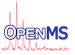OpenMS  2.8.0
EuclideanSimilarity Class Reference

CompareFunctor for 2Dpoints. More...

`#include <OpenMS/COMPARISON/CLUSTERING/EuclideanSimilarity.h>`

Collaboration diagram for EuclideanSimilarity:
[legend]

## Public Member Functions

EuclideanSimilarity ()
default constructor More...

EuclideanSimilarity (const EuclideanSimilarity &source)
copy constructor More...

virtual ~EuclideanSimilarity ()
destructor More...

EuclideanSimilarityoperator= (const EuclideanSimilarity &source)
assignment operator More...

float operator() (const std::pair< float, float > &a, const std::pair< float, float > &b) const
calculates similarity between two points in euclidean space More...

float operator() (const std::pair< float, float > &c) const
calculates self similarity, will yield 0 More...

void setScale (float x)
clusters the indices according to their respective element distances More...

float scale_

## Detailed Description

CompareFunctor for 2Dpoints.

each 2D point as a pair of float holds a float coordinate for each Dimension

## ◆ EuclideanSimilarity() [1/2]

 EuclideanSimilarity ( )

default constructor

## ◆ EuclideanSimilarity() [2/2]

 EuclideanSimilarity ( const EuclideanSimilarity & source )

copy constructor

## ◆ ~EuclideanSimilarity()

 virtual ~EuclideanSimilarity ( )
virtual

destructor

## ◆ operator()() [1/2]

 float operator() ( const std::pair< float, float > & a, const std::pair< float, float > & b ) const

calculates similarity between two points in euclidean space

Parameters
 a a pair of float, giving the x and the y coordinates of the first point b a pair of float, giving the x and the y coordinates of the second point

calculates similarity from the euclidean distance between given 2D points, scaled in [0,1]

setScale

## ◆ operator()() [2/2]

 float operator() ( const std::pair< float, float > & c ) const

calculates self similarity, will yield 0

Parameters
 c a pair of float, giving the x and the y coordinates

## ◆ operator=()

 EuclideanSimilarity& operator= ( const EuclideanSimilarity & source )

assignment operator

## ◆ setScale()

 void setScale ( float x )

clusters the indices according to their respective element distances

Parameters
 x float value to scale the result
Exceptions
 Exception::DivisionByZero if scaling is inapplicable because it is 0

sets the scale so that similarities can be correctly calculated from distances. Should be set so that the greatest distance in a chosen set will be scales to 1 (i.e. `x` = greatest possible distance in the set)

## ◆ scale_

 float scale_
private